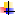# Assignments

## General

There will be three assignments during the semester. First and second ones will cover 30% of the total mark each, and the last third one will cover the rest 40%. Since there is not an examiniation in this subject, total marks of the course will be based on the assignments only.

ASSIGN#
Topic
Allocation
Due
Lecturer
1
Multi-class classificaiotn of English Words using Support Vector Machine (SVM)
30%
JUN. 8th
Dr.Eum
2
Image classification with Tiny imageNet using Convolutional Neural Network (CNN)
30%
JUL. 13th
Dr.Eum
3
Self-regulated open book examination
40%
AUG. 3rd
Dr.EumTraining data set for assignment 1 (HERE)Testing data set for assignment 2 (HERE)

## Questions for assignment 3

#
Question
Topic
Q1
What are the differences between classification and clustering?
Week1
Q2
In which cases is the normal equation good for a linear regression problem (in terms of dimension & size of data)?
Week2
Q3
Why do we use different types of error functions: Mean Square Error and Cross Entropy Error?
Week2
Q4
Discuss the relation between mixing coefficient (𝛑) and responsibility (γ) in a GMM model.
Week3
Q5
Discuss the relation between GMM and HMM based on their corresponding parameters.
Week4
Q6
Why do we introduce the marginalization out (z{k-1}) in the derivation of the solution for viterbi decoding algorithm?
Week4
Q7
What is the phytiscal meaning of lagrange multiplers shown in the dual problem of SVM?
Week5
Q8
Kernel trick: why is it called a trick?
Week5
Q9
What is the difference between XTX and the covariance matrix from cov(X)?
Week6
Q10
Explain the relation between prinicipal components and eign values/vectors of the covariance matrix from cov(X).
Week6
Q11
Explain why cross entropy error function goes well with Softmax activation function.
Week7
Q12
Prove the partial derivative shown in slide 33 (it is related to Q11).
Week8
Q13
What do 1) epoch and 2) batch mean in the training of a machine learning model?
Week9
Q14
Explain the difference between gradient descent (GD) and stochastic gradient descent (SGD).
Week9
Q15
Why is LSTM robust against gradient vanishing problem compared to vanilla RNN?
Week10
Q16
Explain what dropout is and how it can be applied in RNN.
Week11
Q17
Discuss pros and cons of both VAE and GAN models.
Week12
Q18
What is Batch Normalization and why do we use it?
Week13
Q19
Explain why action value fucntion is preferable to state value function in RL.
Week14
Q20
How does experience replay help the convergence of DQN?
Week14
Q21
How does entropy regularization help the exploration in PG based RL algorithms?
Week15
Q22
 Copyright © 2011 OSAKA University - Last update: January 20, 2019, 8:39 pm by Suyong Eum.